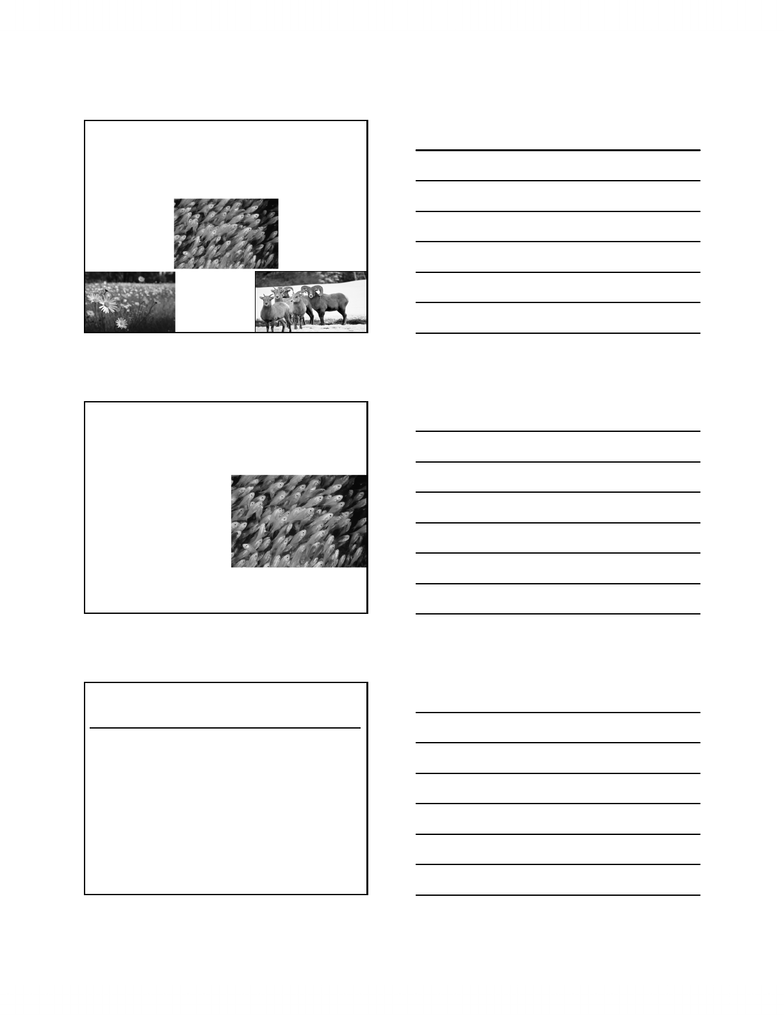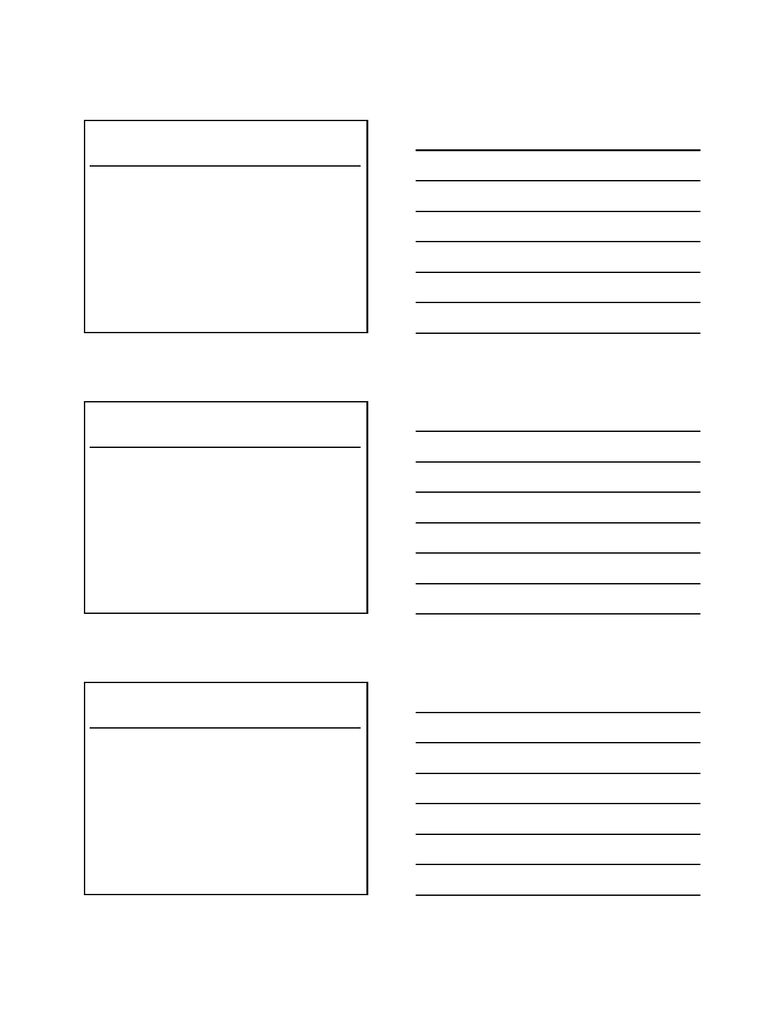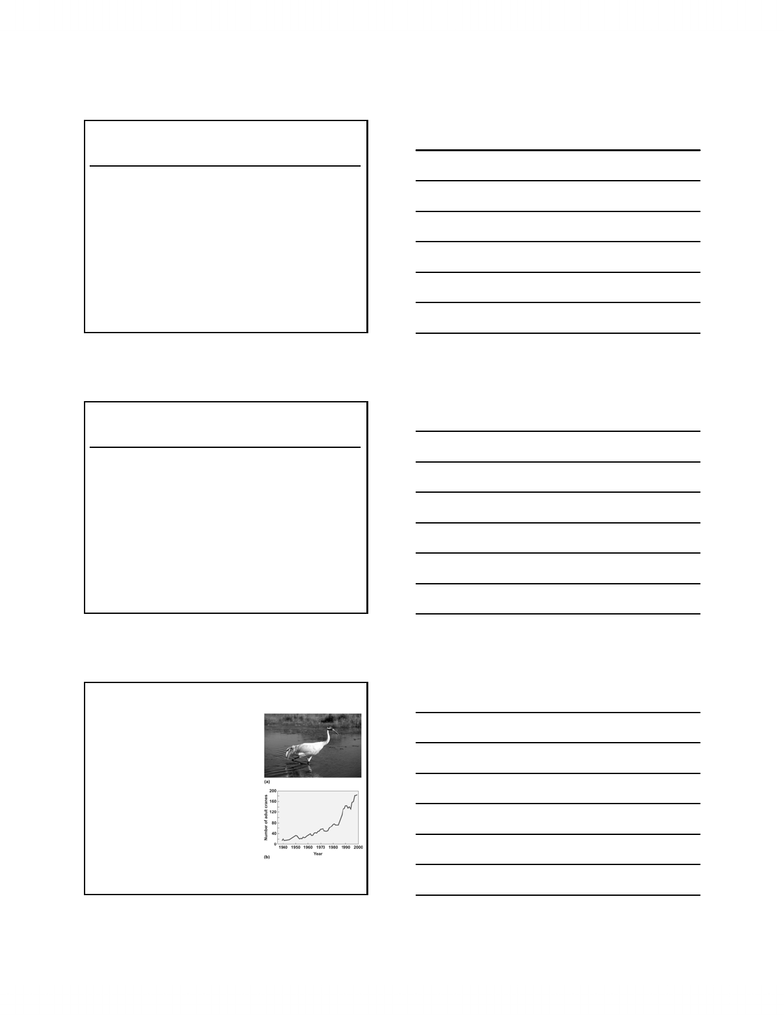# BIO205H5 Lecture Notes - Whooping Crane, Intraspecific Competition, Endangered Species

42 views15 pages
School
UTM
Department
Biology
Course
BIO205H5
ProfessorIntraspecific Population Regulation
Density independent growth
Density dependent growth
Logistic Model
Intraspecific competition
Types of intraspecific competition
Intraspecific competition affects:
- growth/development
- reproduction
-stress
- social behavior
Outline
A) Discrete(geometric)growth
Populationsthatbreedoverdiscretetime
intervals(eg.oneyearperiod)
Allbirthsoccurinspring;doacensusoneyear
laterafterreproductioniscomplete
N(t)=N(0)t
1.DensityIndependentGrowth
- populations increase and discrete growth has time intervals.
- step graph where breeding occurs in time. if birds breed only in may
then they breed, wait till next year and we see how many are breeding.
look at pops from one year to the next.
Unlock document

This preview shows pages 1-3 of the document.
Unlock all 15 pages and 3 million more documents.A) Discreteorgeometricpopulationgrowth
N(t)=N(0)t
N(1)=N(0)1
Eg.,ifnumberoffemalesbreedinginspringof2009=41
Andifnumberoffemalesbreedinginspringof2010=49
=49/41
=1.2
1.DensityIndependentGrowth
B)Exponentialorcontinuouspopulationgrowth
Ifreproductioniscontinuous,thenitismore
appropriatetolookattheinstantaneousrateof
increase=r
Independentoftimeperiods:smoothcurve
N(t)/N(0)=
=erorr=ln
Example:graysquirrelpopulation:r=ln(1.2)=0.18
1.DensityIndependentGrowth
Whatisr?
b=instantaneousbirthrateperindividual
d=instantaneousdeathrateperindividual
dN/dt =(bd)N =rN
dN/dt =rN
r=intrinsicrateofincrease
=rateatwhichapopulationgrowsunderidealconditions
1.DensityIndependentGrowth
-
- not all organisms have a discrete breeding period and have a
continuous period - curve. more appropriate to look at
instantaneous rate of growht (r). we know that a change in
pop can give us lambda and can be rearranged to give us e^r
the ln of which will give us the pop at instantaneous slope.
- use this in life table to figure out the exponential pop growth.
- density independent growth - density has no affect on the growht
so the population has no affect on density and vice versa -
r = birth rate - death rate based on pop size that we have.
Unlock document

This preview shows pages 1-3 of the document.
Unlock all 15 pages and 3 million more documents.“r”
variesamongspecies
variesamongpopulations
dN/dt =(bd)N =rN
Rateofchangeofapopulationovertime,dN/dt,
isafunctionofpopulationsizeN(inrN)
Trend:asyougofromsmalltowardslargeanimals,theintrinsic rateof
increasedeclineswithincreasingsize
PopulationGrowthReflectstheDifferencebetween
RatesofBirthandDeath
WewantanequationtopredictpopulationsizeN(t)under
conditionsofexponentialgrowth
N(t)=N(0)ert
N(t)=numberattimet
N(0)=initialpopulationsizeatt=0
e=baseofnaturallogarithm=2.72
r=intrinsicrateofincreaseinyoung/timeinterval
t=numberoftimeintervals(days,years)
GrowthRatesCanBeUsedtoPredictPopulation
Sizes
Whooping crane
Endangered species: 15 whooping cranes remained in 1941
Calculating Exponential Growth Rate
- Whooping cranes were near extinction due to
overhunting and habitat destruction
Characteristics of population:
-small population size
-protected from hunting
-abundant resources
N = 15 birds in 1941
Q1. If there are 425 birds alive in 2004, what is r?
N(t) =N(0)ert
425 = 15er(63)
425/15 = 28.33 = er(63)
r = ln(28.33)/63
= 3.34/63
= 0.053
- r is not going to be the same among species and among
populations.
- as you go from small to larger animals, the rate of increase
declines with increase in size. a very indicative general trend
that the larger you are for animals, intrinsic rate of ___ declines with
size.
- equation predicts a population size under a certain time under
exponential growth.
- a population is endangered and we can introduce it to an env
that will help it increase in number. take a look at what we can find
out from the data of the population.
- protection laws are put against predators etc and put it in an area
with abundant resources.
- if there are 425 birds in 2004 what is the rate of increase?
- equation of pop at certain period = pop at certain time * e^rt
time difference = 63
-
Unlock document

This preview shows pages 1-3 of the document.
Unlock all 15 pages and 3 million more documents.

## Document Summary

What is r? b = instantaneous birth rate per individual d = instantaneous death rate per individual dn/dt = (b d)n = rn dn/dt = rn r = intrinsic rate of increase. N(t) = n(0)ert: n (t) = number at time t, n(0) = initial population size at t = 0 e = base of natural logarithm = 2. 72 r = intrinsic rate of increase in young/time interval t = number of time intervals (days, years) Whooping cranes were near extinction due to overhunting and habitat destruction. 2 = e(0. 053)t ln 2 = 0. 053t t = 0. 693/0. 053. Endangered species: 15 whooping cranes remained in 1941. Density independent growth: birth and death rates are influenced by density independent factors, regardless of the number of individuals, abiotic factors. The logistic model of population growth dn/dt = rn(1 n/k: when n is low relative to k, the term (1 n/k) is close to.